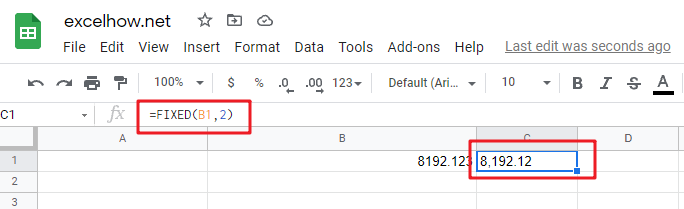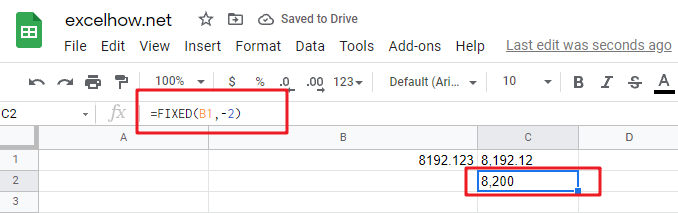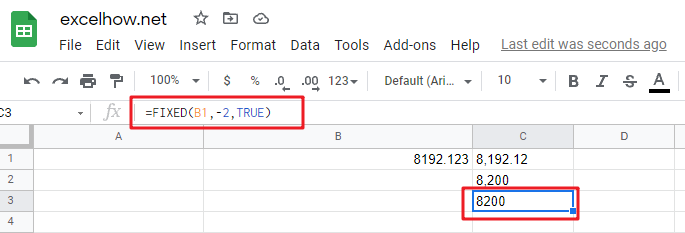# ExcelHow

This post will guide you how to use Google Sheets FIXED function with syntax and examples.

## Description

The Google Sheets FIXED function rounds a number to the specified number of decimal places and returns the result as text string and not a number.

The FIXED function can be used to format a number to text with a fixed number of decimal places in Google Sheets. And it returns a number formatted as text value.

The FIXED function is a build-in function in Google Sheets and it is categorized as a Text Function.

## Syntax

The syntax of the FIXED function is as below:

= FIXED(number, [decimals],[no_commas])

Where the FIXED function arguments are:

• number -This is a required argument. The number to be rounded and converted into text string.
• Decimals -This is an optional argument. It will specify the number of decimal places to be displayed after decimal point.
• No_commas – This is an optional argument.  This is a logical argument. If it is set to TRUE, the result will not separate number groups by commas, or commas are included in the result text string. (If this argument is omitted, the result text string will include commas as default)

Notes:

• If Decimals value is omitted, it is set to be 2 by default.  If Decimals value is negative, the number is rounded to the left of the decimal point.
• The FIXED function can take three arguments, `number`, `decimals` and `no_commas`. `Number` is the number to convert. And Decimals is the number of decimal places which number value should be rounded on the right of the decimal point. If you want to round the left of the decimal point for number, and you can set the decimal value as a negative number.
• The `no_commas` argument is a Boolean value that can be used to control whether commas character should be added to the last text result.
• The result can not be used in a numeric calculation, as it returns as a text value.

## Google Sheets FIXED Function Examples

The below examples will show you how to use Google Sheets FIXED function to round a number to the specified number of decimal places.

#1 To round the number in B1 cell two digits to the right of the decimal point, just using the following FIXED formula:

`= FIXED(B1,2)`#2 To round the number in B2 cell two digits to the left of the decimal point, just using the following formula:

`=FIXED(B1,-2)`#3 To round the number in B3 cell two digit to the left of the decimal point without commas, just using the following formula:

`= FIXED(B1,-2,TRUE)`